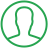# Print Fibonacci Series Using Recursion In Java

Last Updated : Jul 1, 2023In this article we will show you the solution of print fibonacci series using recursion in java, the next number in a Java Fibonacci Series is equal to the sum of the two numbers before it.

The computational run-time analysis of an algorithm to find the greatest common factor of two integers makes extensive use of the Fibonacci numbers.

• Initialized values for previousNumber and nextNumber are 0 and 1, respectively.
• Maximum Number is iterated through via the Fibonacci for Loop.
• Show the prior number
• calculates the sum of the preceding and subsequent numbers.
• new values for previousNumber and nextNumber are updated.

The reasoning is the same as before. The user is required to enter a number rather than having the number of components in the Java Fibonacci Series hardcoded.

Recursive functions are any that have the ability to call themselves.

fibonacciRecursion():

1. An input number is required by the Java Fibonacci recursive function. the Fibonacci numbers in Java begins with 0, 1, 1, therefore it checks for 0,1, 2 and returns 0,1,1 correspondingly.
2. The function will recursively call itself when the input n is greater than 3. The call is placed twice. Using a recursion example with a 4 as the input, let's examine a Fibonacci Series in Java.

A Fibonacci sequence is a group of numbers where each number (aside from the first two) equals the sum of a two before it.

Iteration or recursion can be used in Java to generate the Fibonacci sequence. This article will discuss the Fibonacci series utilizing Java recursion.

In addition to the first two numbers, every number in a Fibonacci series seems to be the product of the two numbers that came before it.

A Fibonacci series has the mathematical formula F(n)=F(n1)+F(n2)

## Step By Step Guide On Print Fibonacci Series Using Recursion In Java :-

```public class TalkersCode{
public static void main(String[] args)
{
int maxNo = 5;
int previousNo = 0;
int nextNo = 1;
System.out.print("Fibonacci Series of "+maxNo+" numbers:");
for (int i = 1; i <= maxNo; ++i)
{
System.out.print(previousNo+" ");
int sum = previousNo + nextNo;
previousNo = nextNo;
nextNo = sum;
}
}
}```
1. We first make a class called TalkersCode.
2. Every number of elements inside the Fibonacci Series is then set to the number you desire.
3. Then we generate integer numbers for the maximum, preceding, and subsequent values.
4. Finally, we define the second number is given the first number on each iteration, and the second number is given the sum of the previous two numbers.
5. The programme is then close.

## Conclusion :-

In order to conduct recursion when computing the Fibonacci Series in Java, a function must first be created.

The input for this function is an integer. The function determines whether the input is 0 or 1, 1, or 2, and if it is any one of those values, it returns 0 or 1 (for the second Fibonacci), correspondingly.

If the input is larger than 2, this function runs itself repeatedly for the prior values up until the input variable's value drops to less or equal to 2.

I hope this article on print fibonacci series using recursion in java helps you and the steps and method mentioned above are easy to follow and implement.

##About Dikshita

Passionate Electronics and Communication Engineering student with expertise in web development (HTML, CSS, JS,PHP, Bootstrap, React.js) and content writing. Eager problem solver and tech enthusiast, adept at creating engaging web experiences.